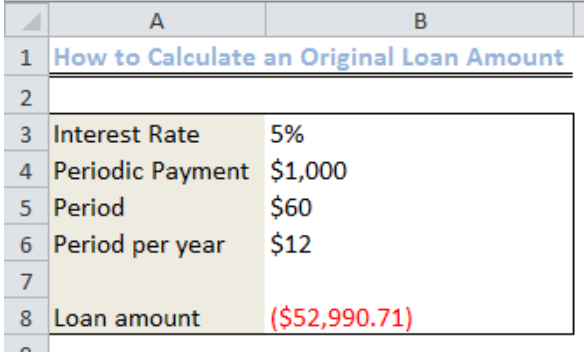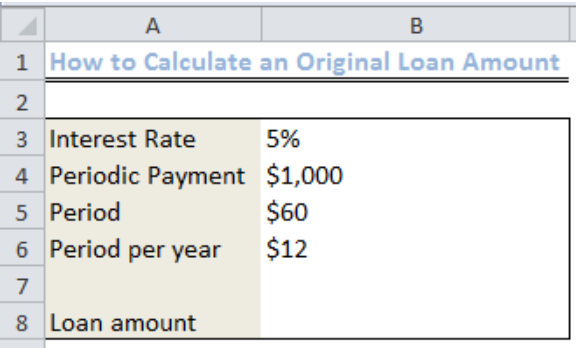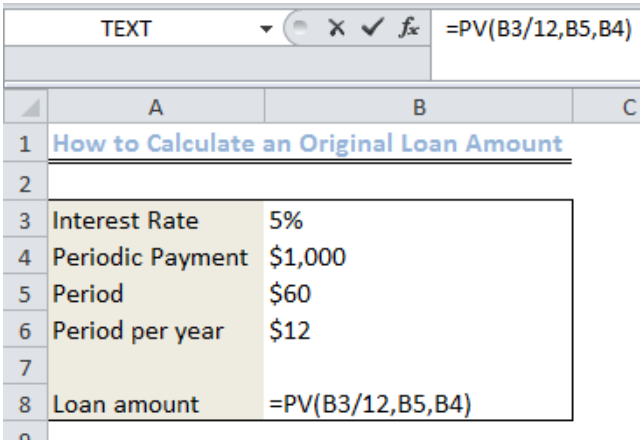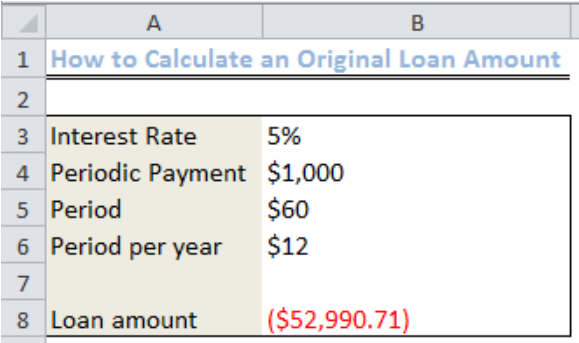Get instant live expert help with Excel or Google Sheets“My Excelchat expert helped me in less than 20 minutes, saving me what would have been 5 hours of work!”

#### Post your problem and you'll get expert help in seconds

Your message must be at least 40 characters
Our professional experts are available now. Your privacy is guaranteed.

# How to Calculate an Original Loan Amount in Excel

We can calculate an original loan amount by using the Present Value Function (PV) if we know the interest rate, periodic payment, and the given loan term. This function tells the present value of an investment.The steps below will walk you through the process of calculating an original loan amount.Figure 1: Original Loan Amount

## Setting up the Data

We will input the values as shown in figure 2 into Column A and Column BFigure 2: Data to Calculate an Original Loan Amount

## Syntax

`PV(rate, nper, pmt)`

## Explanation

• Rate

The rate is calculated as the interest rate per period. If we collectively obtain a loan at a 15% annual interest and make monthly payments, the interest rate per month is 15%/12 or 0.0125. We can input any of the following as the rate:

• 0.0125
• The cell containing the interest rate divided by 12
• 15%/12
• Nper

This is the total number of payments we will make for the loan. Assuming we are to pay the loan monthly in four years, the period is 4*12 (48) periods. We will input this value into the formula.

• Pmt

This is the amount we will pay for each month within the four-year period. This amount covers only the principal which we collected and the interest.

• Formula

`=PV(B3/12,B5,B4)`

We will type or copy and paste this formula into Cell B8.Figure 3: Inserting the Formula to Calculate the Original Loan Amount

We will now press ENTERFigure 4: Result of the Original Loan Amount

## Instant Connection to an Expert through our Excelchat Service

Most of the time, the problem you will need to solve will be more complex than a simple application of a formula or function. If you want to save hours of research and frustration, try our live Excelchat service! Our Excel Experts are available 24/7 to answer any Excel question you may have. We guarantee a connection within 30 seconds and a customized solution within 20 minutes.

### Did this post not answer your question? Get a solution from connecting with the expert.Another blog reader asked this question today on Excelchat:## Subscribe to Excelchat.coAnother blog reader asked this question today on Excelchat: